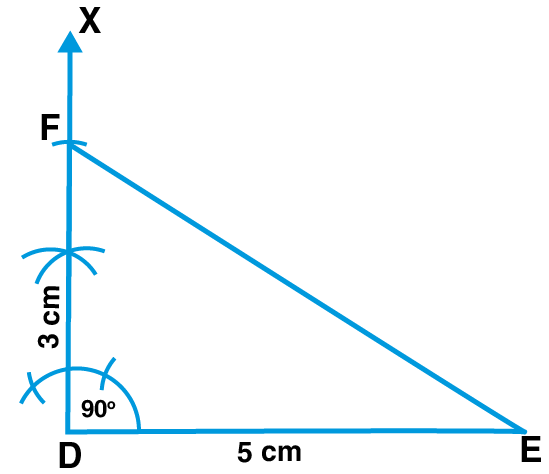# ML Aggarwal Solutions for Class 7 Maths Chapter 13 Practical Geometry

ML Aggarwal Solutions for Class 7 Maths Chapter 13 Practical Geometry consists of answers designed by expert teachers at BYJU’S. The solutions clear doubts of students instantly, which arise while practicing textbook questions. Continuous practice of ML Aggarwal Solutions boosts confidence in solving complex problems with ease. Students can cross check their answers while revising exercise problems before the final examination. This makes them realize the percent of knowledge obtained about the concepts covered. For more details, students are suggested to follow ML Aggarwal Solutions for Class 7 Maths Chapter 13 Practical Geometry PDF, from the links available below

Chapter 13 deals with the study of construction of parallel lines to a line, through a point not on the line and various topics on Practical Geometry. These solutions help students to improve problem solving and time management skills, which is vital for exam preparation.

## ML Aggarwal Solutions for Class 7 Maths Chapter 13 Practical Geometry Download PDF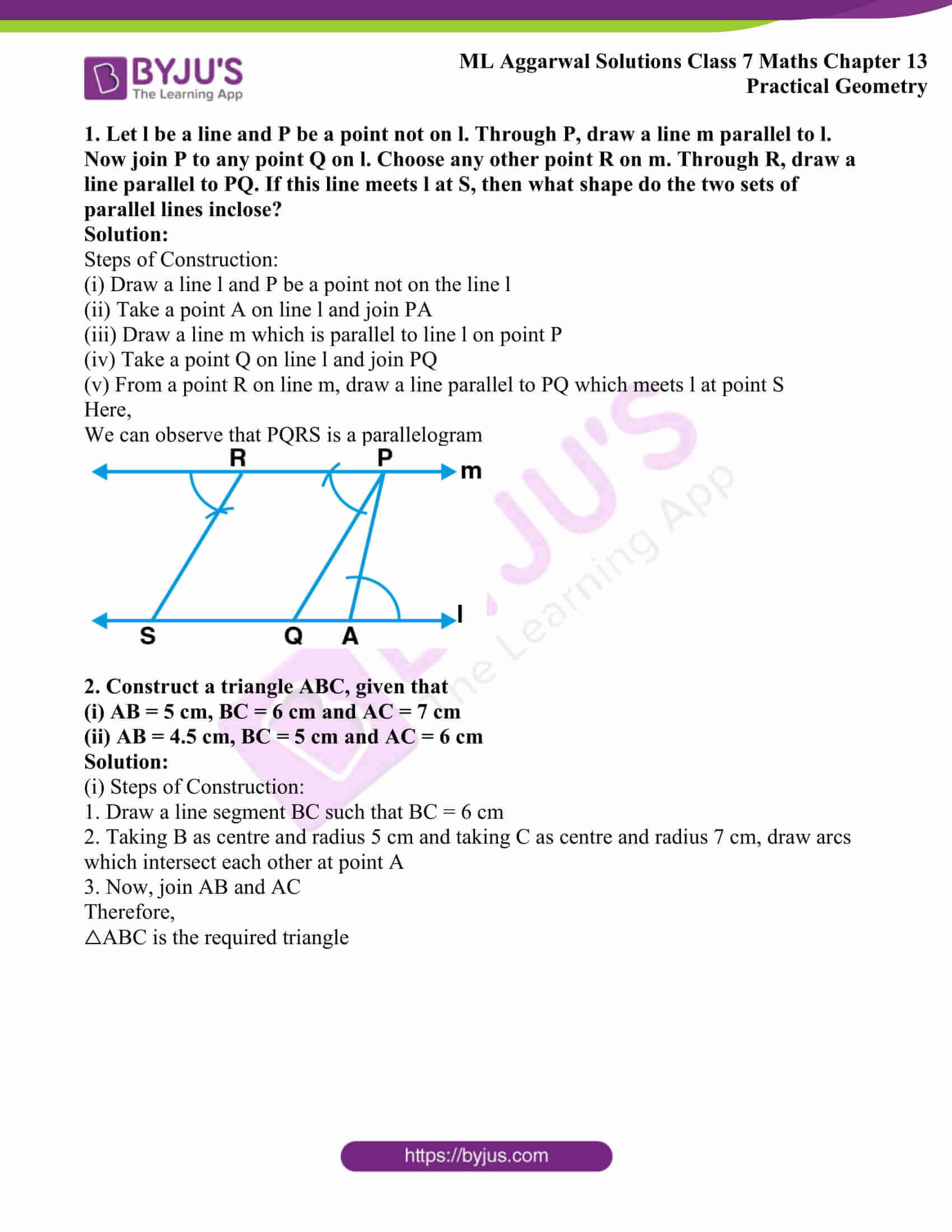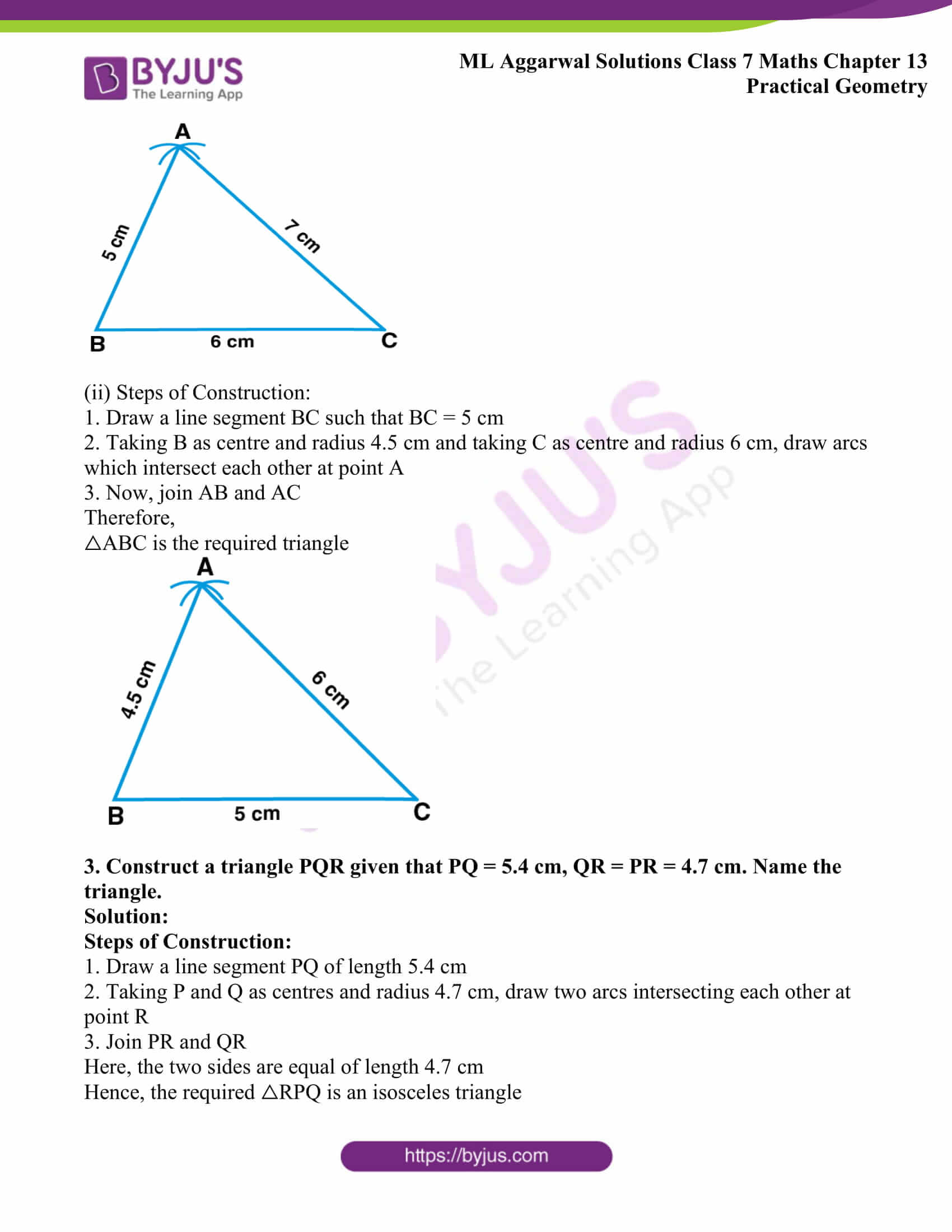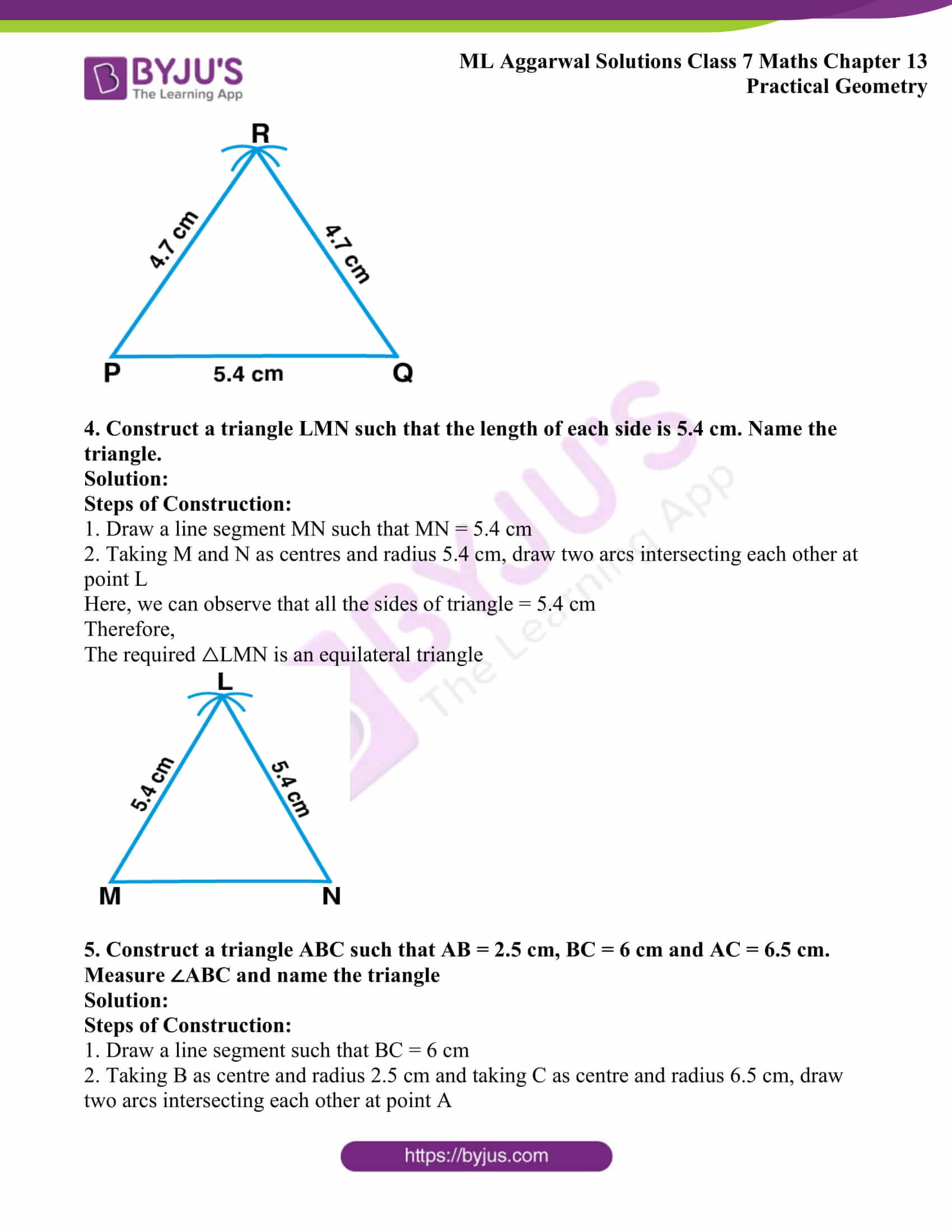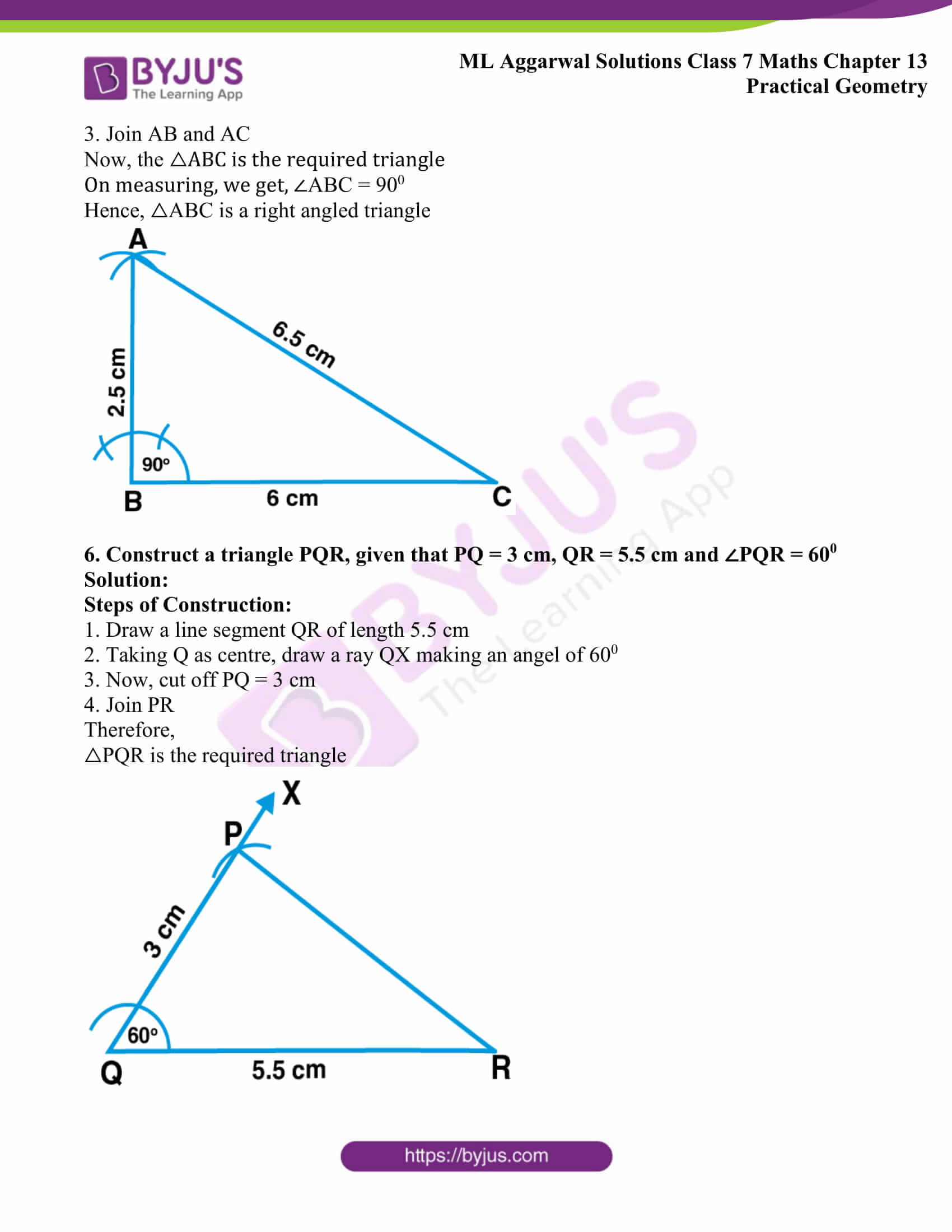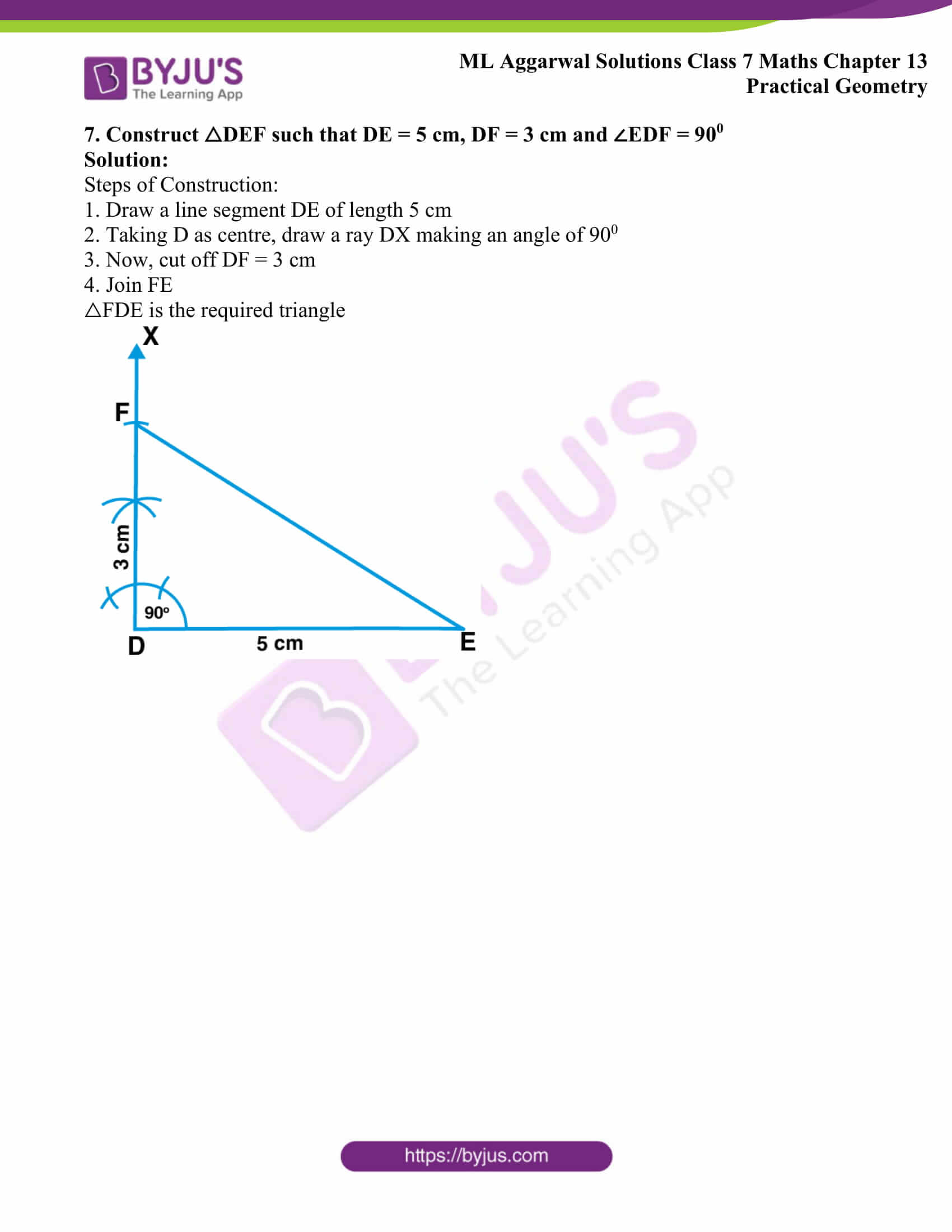## Access ML Aggarwal Solutions for Class 7 Maths Chapter 13 Practical Geometry

1. Let l be a line and P be a point not on l. Through P, draw a line m parallel to l. Now join P to any point Q on l. Choose any other point R on m. Through R, draw a line parallel to PQ. If this line meets l at S, then what shape do the two sets of parallel lines inclose?

Solution:

Steps of Construction:

(i) Draw a line l and P be a point not on the line l

(ii) Take a point A on line l and join PA

(iii) Draw a line m which is parallel to line l on point P

(iv) Take a point Q on line l and join PQ

(v) From a point R on line m, draw a line parallel to PQ which meets l at point S

Here,

We can observe that PQRS is a parallelogram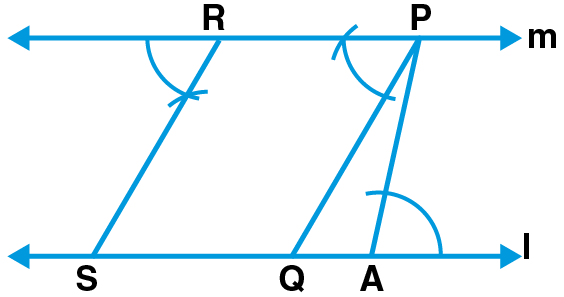2. Construct a triangle ABC, given that

(i) AB = 5 cm, BC = 6 cm and AC = 7 cm

(ii) AB = 4.5 cm, BC = 5 cm and AC = 6 cm

Solution:

(i) Steps of Construction:

1. Draw a line segment BC such that BC = 6 cm

2. Taking B as centre and radius 5 cm and taking C as centre and radius 7 cm, draw arcs which intersect each other at point A

3. Now, join AB and AC

Therefore,

△ABC is the required triangle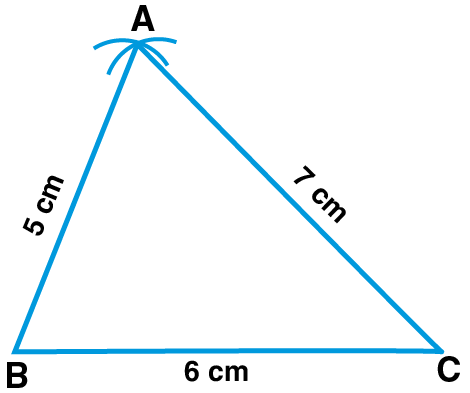(ii) Steps of Construction:

1. Draw a line segment BC such that BC = 5 cm

2. Taking B as centre and radius 4.5 cm and taking C as centre and radius 6 cm, draw arcs which intersect each other at point A

3. Now, join AB and AC

Therefore,

△ABC is the required triangle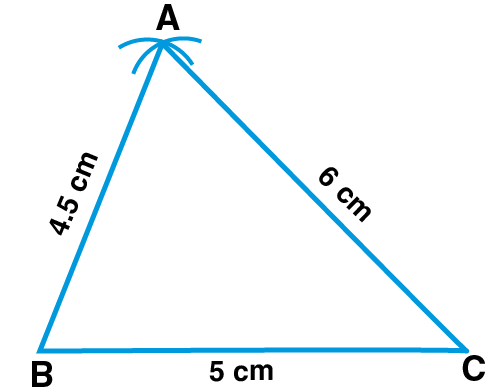3. Construct a triangle PQR given that PQ = 5.4 cm, QR = PR = 4.7 cm. Name the triangle.

Solution:

Steps of Construction:

1. Draw a line segment PQ of length 5.4 cm

2. Taking P and Q as centres and radius 4.7 cm, draw two arcs intersecting each other at point R

3. Join PR and QR

Here, the two sides are equal of length 4.7 cm

Hence, the required △RPQ is an isosceles triangle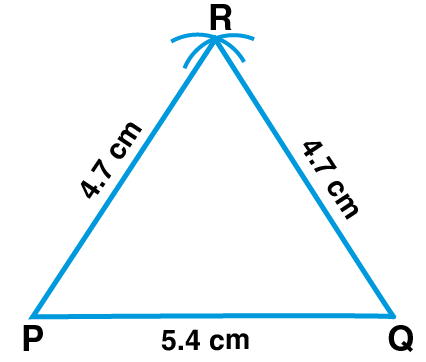4. Construct a triangle LMN such that the length of each side is 5.4 cm. Name the triangle.

Solution:

Steps of Construction:

1. Draw a line segment MN such that MN = 5.4 cm

2. Taking M and N as centres and radius 5.4 cm, draw two arcs intersecting each other at point L

Here, we can observe that all the sides of triangle = 5.4 cm

Therefore,

The required △LMN is an equilateral triangle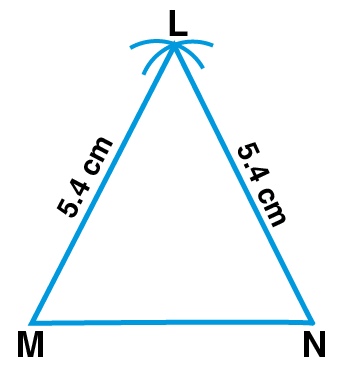5. Construct a triangle ABC such that AB = 2.5 cm, BC = 6 cm and AC = 6.5 cm. Measure ∠ABC and name the triangle

Solution:

Steps of Construction:

1. Draw a line segment such that BC = 6 cm

2. Taking B as centre and radius 2.5 cm and taking C as centre and radius 6.5 cm, draw two arcs intersecting each other at point A

3. Join AB and AC

Now, the △ABC is the required triangle

On measuring, we get, ∠ABC = 900

Hence, △ABC is a right angled triangle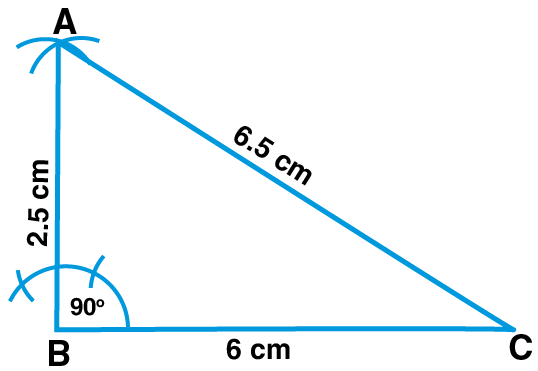6. Construct a triangle PQR, given that PQ = 3 cm, QR = 5.5 cm and ∠PQR = 600

Solution:

Steps of Construction:

1. Draw a line segment QR of length 5.5 cm

2. Taking Q as centre, draw a ray QX making an angel of 600

3. Now, cut off PQ = 3 cm

4. Join PR

Therefore,

△PQR is the required triangle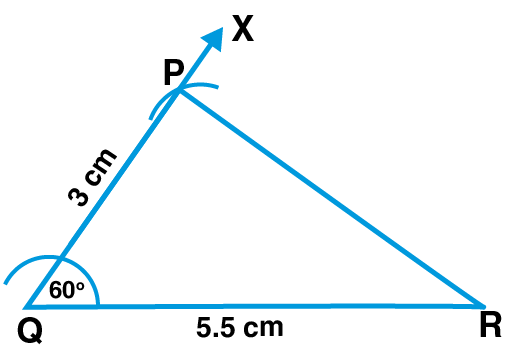7. Construct △DEF such that DE = 5 cm, DF = 3 cm and ∠EDF = 900

Solution:

Steps of Construction:

1. Draw a line segment DE of length 5 cm

2. Taking D as centre, draw a ray DX making an angle of 900

3. Now, cut off DF = 3 cm

4. Join FE

△FDE is the required triangle